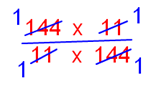# Properties of Fractional Division

Properties of fractional division are discussed here:

Property 1: When a fractional number is divided by 1, the quotient is the fractional number itself.

For Example:

1. 2/3 ÷ 1

= 2/3 × 1/1

= (2 × 1)/(3 × 1)

= 2/3

2. 11/16 ÷ 1

= 11/16 × 1/1

= (11 × 1)/(16 × 1)

= 11/16

3. 21/43 ÷ 1

= 21/43 × 1/1

= (21 × 1)/(43 × 1)

= 21/43

Property 2:When zero is divided by non zero fractional number, then the quotient is always zero.

For Example:

1. 0 ÷ 7/9

= 0 × 9/7

= 0

2. 0 ÷ 18/33

= 0 × 33/18

= 0

3. 0 ÷ 29/16

= 0 × 16/29

= 0

Property 3: When any non zero fractional number is divided by itself, then the quotient is 1.

For Example:

1. 19/21 ÷ 19/21

= 19/21 × 21/19= 1

2. 144/11 ÷ 144/11

= 144/11 × 11/144= 1

Property 4: The reciprocal of zero or multiplicative inverse does not exist. So a fractional number cannot be divided by zero (0).